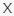# Christmas Picture Puzzle Quiz VideoQuizStar

Q 1. Let’s start! Which cool penguin is different from the others?Choices:

A
B
C

B

Q 2. Think you can spot the snowman that is the odd one out?Choices:

A
B
C

C

Q 3. Ho Ho Ho! Which picture is different?Choices:

A
B
C

C

Q 4. Can you figure out which reindeer is different?Choices:

A
B
C

B

Q 5. Which colorful arrangement of Christmas hats is the odd one out?Choices:

A
B
C
D

B

Q 6. Can you spot the odd one out?Choices:

A
B
C
D

B

Q 7. Which picture is different from the others?Choices:

A
B
C
D

C

Q 8. Take a look at these gingerbread cookies! Think you can figure out which picture is different?Choices:

A
B
C
D

B

##### Attention to Question 9, There are 2 random photos, if you have different picture, then refresh your quiz link until you find picture like in this post!

Q 9. Which two Christmas trees are identical?Choices:

1 and 5
3 and 4
2 and 5
1 and 4

1 and 5

Q Alt 9. Which two Christmas trees are identical?Choices:

1 and 5
3 and 4
2 and 5
1 and 4

3 and 4

##### Attention to Question 10, There are 3 random photos, if you have different picture, then refresh your quiz link until you find picture like in this post!

Q 10. Which two gifts are identical?Choices:

1 and 5
2 and 4
4 and 7
1 and 6
3 and 5

3 and 5

Q Alt 10. Which two gifts are identical?Choices:

1 and 5
3 and 4
4 and 7
1 and 6
3 and 5

3 and 4

Q Alt 10-2. Which two gifts are identical?Choices:

4 and 6
7 and 7
2 and 4
1 and 5
1 and 3

1 and 3

Q 11. Think you can figure out which silhouette matches exactly with the reindeer on the left?Choices:

A
B
C
D

C

##### Attention to Question 12, There are 3 random photos, if you have different picture, then refresh your quiz link until you find picture like in this post!

Q 12. Take a close look here! Which silhouette matches exactly with the image of Santa on the left?Choices:

A
B
C
D

A

Q Alt 12. Take a close look here! Which silhouette matches exactly with the image of Santa on the left?Choices:

A
B
C
D

B

Q Alt 12-2. Take a close look here! Which silhouette matches exactly with the image of Santa on the left?Choices:

A
B
C
D

C

##### Attention to Question 13, There are 3 random photos, if you have different picture, then refresh your quiz link until you find picture like in this post!

Q 13. Which silhouette matches exactly with the collection of toys on the left?Choices:

A
B
C
D

A

Q Alt 13. Which silhouette matches exactly with the collection of toys on the left?Choices:

A
B
C
D

B

Q Alt 13-2. Which silhouette matches exactly with the collection of toys on the left?Choices:

A
B
C
D

D

Q 14. Which delicious Christmas treat is NOT in the image?Choices:

A
B
C
D

D

Q 15. Click PLAY to watch the video and identify which two snowflakes are exactly the same!Choices:

A
B
C
D
E